Function Repository Resource:

# NestedLookup

Look up a set of keys in order to get deeper parts of an association or list of rules

Contributed by: Richard Hennigan (Wolfram Research)
 ResourceFunction["NestedLookup"][assoc,{key1,key2,…}] looks up the value associated with the keys key1,key2,… in deeper levels of assoc. ResourceFunction["NestedLookup"][assoc,keys, default] gives default if the part specified by keys is not found. ResourceFunction["NestedLookup"][{key1,key2,…}] represents an operator form of ResourceFunction["NestedLookup"] that can be applied to an expression.

## Details and Options

ResourceFunction["NestedLookup"][assoc,{key1,key2,}] is effectively equivalent to Fold[Lookup,assoc,{key1,key2,}] but returns Missing["KeySequenceAbsent",keys] if the specified part is not found.
In ResourceFunction["NestedLookup"][assoc,keys,default], default is only evaluated if the key sequence keys has any part missing.
ResourceFunction["NestedLookup"] can be applied to expressions that are Association objects or lists of rules at each level.
ResourceFunction["NestedLookup"][assoc,{,Key[list],}] treats the list list as a single key rather than a list of keys.
ResourceFunction["NestedLookup"][{key1,key2,}][assoc] is equivalent to ResourceFunction["NestedLookup"][assoc,{key1,key2,}].

## Examples

### Basic Examples (4)

Retrieve the ResourceFunction:

 In:=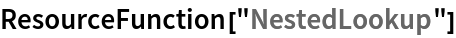Out=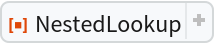Look up a nested part of an association:

 In:=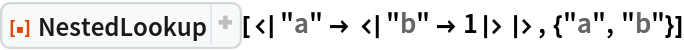Out=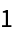In:=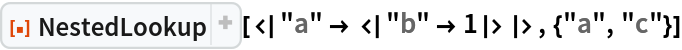Out=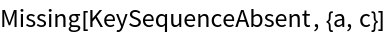When a third argument is provided and the key sequence has a part missing, the default value is returned:

 In:=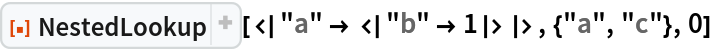Out=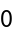### Scope (5)

When the key sequence is present, the default is not evaluated:

 In:=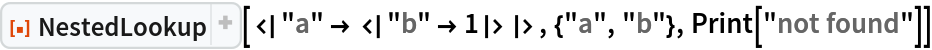Out=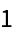NestedLookup threads over lists of associations:

 In:=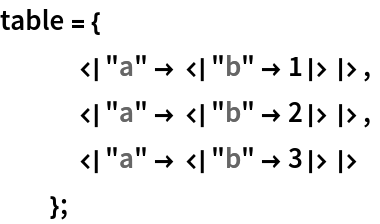In:=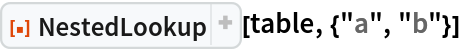Out=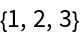NestedLookup can be used to look up keys in lists of rules:

 In:=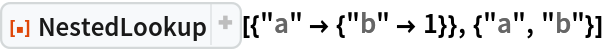Out=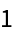Lists of rules can be mixed with Associations:

 In:=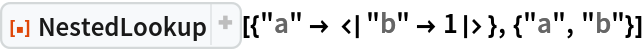Out=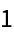Use the operator form of NestedLookup:

 In:=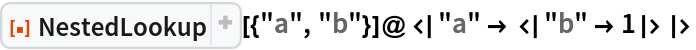Out=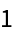### Applications (1)

You can use constructs such as Throw and Return in the third argument of NestedLookup to abort a computation when a required key is missing:

 In:=In:=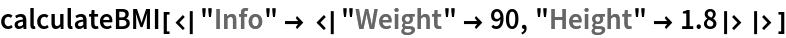Out=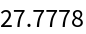In:=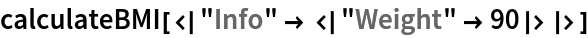Out=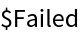### Properties and Relations (3)

Applying an Association to a sequence of keys is typically equivalent to using NestedLookup on a list of those keys:

 In:=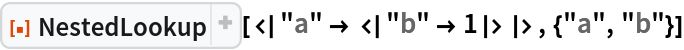Out=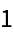In:=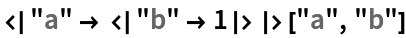Out=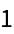Lists are handled differently by NestedLookup, however:

 In:=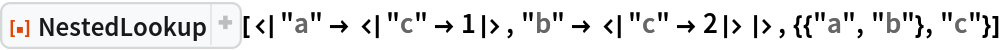Out=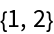In:=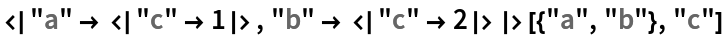Out=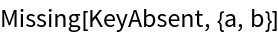Wrap the list in Key to make NestedLookup treat it as a single key:

 In:=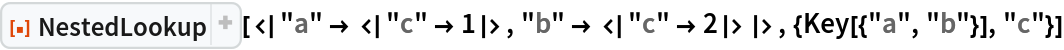Out=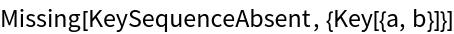### Possible Issues (2)

When the key to be looked up is a list or an expression with head Key, it must be wrapped in Key to avoid ambiguity:

 In:=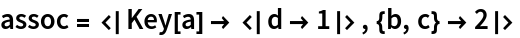Out=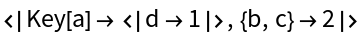In:=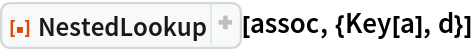Out=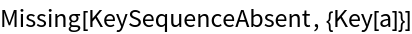In:=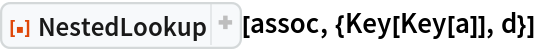Out=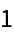In:=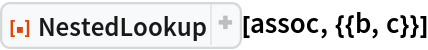Out=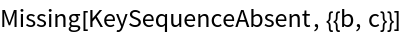In:=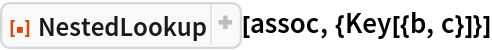Out=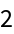When a part is not found, NestedLookup only reports the sequence up to the missing key:

 In:=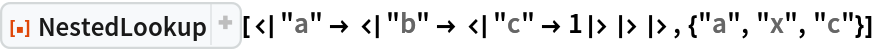Out=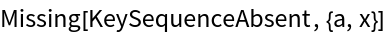## Requirements

Wolfram Language 11.3 (March 2018) or above

## Version History

• 1.0.0 – 12 October 2018